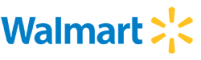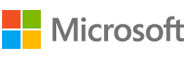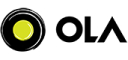New update is available. Click here to update.
Last Updated: 6 Dec, 2020

# 0 1 Knapsack

Easy+37 more companies

## Problem statement#### A thief is robbing a store and can carry a maximal weight of W into his knapsack. There are N items and the ith item weighs wi and is of value vi. Considering the constraints of the maximum weight that a knapsack can carry, you have to find and return the maximum value that a thief can generate by stealing items.

##### Input Format:
``````The first line contains a single integer T representing the number of test cases.
The T-test cases are as follows:

Line 1:The first line contains an integer, that denotes the value of N.
Line 2:The following line contains N space-separated integers, that denote the values of the weight of items.
Line 3:The following line contains N space-separated integers, that denote the values associated with the items.
Line 4:The following line contains an integer that denotes the value of W. W denotes the maximum weight that a thief can carry.
``````
##### Output Format :
``````The first and only line of output contains the maximum value that a thief can generate, as described in the task.
The output of every test case is printed in a separate line.
``````
##### Constraints:
``````1 <= T <= 10
1 <= N <= 10^2
1<= wi <= 50
1 <= vi <= 10^2
1 <= W <= 10^3

Time Limit: 1 second
``````
``````Can we solve this using space complexity of not more than O(W)?
``````## Approaches

### 01 ApproachApproach: In the Dynamic programming we will work considering the same cases as mentioned in the recursive approach. In a DP[][] table let’s consider all the possible weights from ‘1’ to ‘W’ as the columns and weights that can be kept as the rows.
The state DP[i][j] will denote maximum value of ‘j-weight’ considering all values from ‘1 to ith’. So if we consider ‘wi’ (weight in ‘ith’ row) we can fill it in all columns which have ‘weight values > wi’. Now two possibilities can take place:

• Fill ‘wi’ in the given column.
• Do not fill ‘wi’ in the given column.

Now we have to take a maximum of these two possibilities, formally if we do not fill ‘ith’ weight in ‘jth’ column then DP[i][j] state will be same as DP[i-1][j] but if we fill the weight, DP[i][j] will be equal to the value of ‘wi’+ value of the column weighing ‘j-wi’ in the previous row. So we take the maximum of these two possibilities to fill the current state. This visualization will make the concept clear: